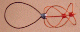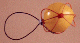# Proton 1: 2nd Degree (P1-21S)The Proton Shell of 1 is an un-filled shell built from two hadron strings. The equatorial string is in red. The sinusoidal string goes twice around the particle to close on itself, it is the orange string. The Pinned Vector Boson (PVB) is a lepton string pinned to one of the proton's three Up quarks to emulate the properties of a Down quark, this type of quark is referred to as a Bogus Down quark. The lepton string is shown in black, it contributes one snap point and a charge of -1. (The yellow balloon is used to hide the background strings for a less confusing view.) The model pictured was made with a 7.5" and a 18" length of wire. All the models with unknown amplitude sinusoidal strings have been recalculated to show the maximum length of the sinusoidal string based on a harmonic potential of the geometry. See Mensuration: Sinusoidal. This is also the structural model of the muon residing in lepton space. In the case of the muon, the core geometry is composed of lepton string rather than hadron string. The muon pins a hadron string, resulting in an electron with elevated mass. (The hadron string participates in hadron gravitational interactions, whereas the electron is composed completely of lepton string, permitting only lepton gravitational interactions.) Once the muon sheds the radical hadron string it will pin a lepton string to replace it, turning it into a conventional electron with an orphan string.

## Stereograms of Proton (Upper Right) and Neutron ShellThe lower left and lower right shells represent the neutron, on the left the neutron is shown with two seperate P.V.B.'s, and on the right it is shown with a single doubly-pinned P.V.B.. Note that the core geometry of the proton and neutron are the same, they vary only in the number of pinning sites.

 Required Snap Points: 10 Available Snap Points: 9 Pinned Vector Bosons: 1 *L1 = 14.17424118" (Equatorial) *L2 = 36.82575882" (Sinusoidal) Model Diameter: 4.511801096" *Loop Ratio (1:2): 0.384900179 * (Extrapolated Dimensions) Loop Equation: 1(L1) + 1(L2) = 51" Snap Point Equation: 1(3) + 1(6) = 9 A.S.P. PVB's:(+ 1 P.V.B. = 10 R.S.P.)

Copyright 1997 by Arnold J. Barzydlo
Go Back